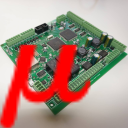#myCNC Online Documentation

### Site Tools

plc:m88_m89_stop_motion_from_plc_if_input_pin_activated

## M88, M89 PLC procedures. Stop Motion if Input pin activated

Video recap of the manual is available here:

One of the most popular job for the PLC procedure is moving to given direction till input pin triggered.

It's used in

• Homing procedures,
• Probing,
• Tool Length measure
• Surface measure
• Gantry Alignment procedure
• and many others

This procedure can be handled in Hardware PLC. We offer M88 and M89 PLC procedures which do this job as a standard procedure. However, it can be customized easily according to customer needs.

A source code for M88 PLC procedure is shown below

M88.plc
```main ()
{

input=eparam&0xFFFF;	//P-parameter
state=eparam>>16;	//L-parameter

message=PLCCMD_MOTION_CONTINUE;
timer=30;do{timer--;}while(timer>0);

ready=0;

do {
a=portget(input);
if (state==0)
{
if (a==0) {ready=1;};
};
if (state!=0)
{
if (a!=0) {ready=1;};
};
}while(ready==0);

message=PLCCMD_MOTION_SOFT_SKIP;
timer=30;do{timer--;}while(timer>0);

exit(99);
};```

Input pin number and pins state (normally opened/closed) comes from G-code P/L parameters in eparam internal variable. Pin number comes in P-parameter and pin state in L-parameter.

• L=0 means the procedure waits logical “0” on selected input pin
• L=1 means the procedure waits logical “1” on selected input pin

Example:

```M88 P7 L0 (The procedure will wait "0" level on input pin #7)
G90 G0X-1000 F300```
```M88 P3 L1 (The procedure will wait "1" level on input pin #3)
G90 G0Y300 F100```

A two-lines block to decode Input pin number and a pin state from eparam variable is shown below

M88.plc
```  input=eparam&0xFFFF;	//P-parameter
state=eparam>>16;	//L-parameter```

After input pin number and state is decoded, the PLC procedure sends to the Motion controller a command to start next G-code line which is supposed to be a G0-positioning line.

```  message=PLCCMD_MOTION_CONTINUE;
timer=30;do{timer--;}while(timer>0);```

Then the PLC procedure tests selected pin and is waiting till the pin comes to the given state (depends on L/state parameter) The test code is wrapped in do{}while; loop.

```  ready=0;

do {
a=portget(input);
if (state==0)
{
if (a==0) {ready=1;};
};
if (state!=0)
{
if (a!=0) {ready=1;};
};
}while(ready==0);```

After the pin came to the given state, the PLC sends to the Motion controller to skip the current motion command and load the next.

```  message=PLCCMD_MOTION_SOFT_SKIP;
timer=30;do{timer--;}while(timer>0);```

There are 2 scenarios how to Stop the current motion.

1. Immediate STOP (abort pulses generation right away)
2. Soft STOP (do soft deceleration with given deceleration time, programmed as Soft stop time, s in Configuration settings of the myCNC control software.

If motion speed is low and you need to find a precise position of input pin triggered you would need Immediate STOP. However, if motion speed is high and you need to find the first estimate, Immediate STOP would be harmful for machine mechanics and Soft STOP might be more preferable.

The Immediate of Soft stop can be choosen by sending PLC message to the Motion Controller

Message Code Value Description
PLCCMD_MOTION_SOFT_SKIP 1003 Soft stop and jump to the next command
PLCCMD_MOTION_SKIP 1002 Immediate stop and kump to the next command

M88.plc procedure does Soft Stop if a input pin activated. M89.plc procedure does Immediate Stop if a input pin activated.

The only difference between M88 and M89 procedures are message to the Motion controller to skip current motion.

for M89.plc the code is

```  message=PLCCMD_MOTION_SKIP;
timer=30;do{timer--;}while(timer>0);```

A complete source for M89.plc is shown below

M89.plc
```main ()
{

input=eparam&0xFFFF;	//P-parameter
state=eparam>>16;	//L-parameter

message=PLCCMD_MOTION_CONTINUE;
timer=30;do{timer--;}while(timer>0);

ready=0;

do {
a=portget(input);
if (state==0)
{
if (a==0) {ready=1;};
};
if (state!=0)
{
if (a!=0) {ready=1;};
};
}while(ready==0);

message=PLCCMD_MOTION_SKIP;
timer=30;do{timer--;}while(timer>0);

exit(99);
};```
plc/m88_m89_stop_motion_from_plc_if_input_pin_activated.txt · Last modified: 2021/01/13 12:52 by ivan

### Page Tools